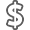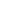# Numerical Analysis.

• ISBN13:

• ISBN10:

## 013469645X

• Edition: 3rd
• Format: Hardcover
• Publisher: Pearson

Note: Supplemental materials are not guaranteed with Rental or Used book purchases.

Purchase Benefits

•Free Shipping On Orders Over \$35!
Your order must be \$35 or more to qualify for free economy shipping. Bulk sales, PO's, Marketplace items, eBooks and apparel do not qualify for this offer.
•Get Rewarded for Ordering Your Textbooks! Enroll Now
•We Buy This Book Back!
In-Store Credit: \$47.77
Check/Direct Deposit: \$45.50
PayPal: \$45.50
List Price: \$193.32 Save up to \$143.83
• Rent Book \$115.99Free Shipping

TERM
PRICE
DUE
CURRENTLY AVAILABLE, USUALLY SHIPS IN 24-48 HOURS
*This item is part of an exclusive publisher rental program and requires an additional convenience fee. This fee will be reflected in the shopping cart.

### Supplemental Materials

What is included with this book?

• The New copy of this book will include any supplemental materials advertised. Please check the title of the book to determine if it should include any access cards, study guides, lab manuals, CDs, etc.
• The Rental and eBook copies of this book are not guaranteed to include any supplemental materials. Typically, only the book itself is included. This is true even if the title states it includes any access cards, study guides, lab manuals, CDs, etc.

### Summary

For courses in Numerical Analysis.

Helps students construct and explore algorithms for solving science and engineering problems

Numerical Analysis, 3rd Edition is for students of engineering, science, mathematics, and computer science who have completed elementary calculus and matrix algebra. It covers both standard topics and some of the more advanced numerical methods used by computational scientists and engineers, while remaining readable and relevant for undergraduates.

Sauer discusses the fundamental concepts of numerical analysis: convergence, complexity, conditioning, compression, and orthogonality. Throughout, Spotlight features comment on each of these concepts as they are addressed, and make connections to other expressions of the same principle elsewhere in the book. The popular Reality Check in each chapter gives concrete, relevant examples of the way numerical methods lead to solutions of important scienti c and technological problems; they can be used to launch individual or team projects. MATLAB® is used throughout to demonstrate and implement numerical methods.

013469645X / 9780134696454  Numerical Analysis, 3/e

### Author Biography

Timothy Sauer earned his Ph.D. in mathematics at the University of California–Berkeley in 1982, and is currently a professor at George Mason University. He has published articles on a wide range of topics in applied mathematics, including dynamical systems, computational mathematics, and mathematical biology.

CHAPTER 0      Fundamentals

0.1 Evaluating a Polynomial

0.2 Binary Numbers

0.2.1 Decimal to binary

0.2.2 Binary to decimal

0.3 Floating Point Representation of Real Numbers

0.3.1 Floating point formats

0.3.2 Machine representation

0.3.3 Addition of floating point numbers

0.4 Loss of Significance

0.5 Review of Calculus

CHAPTER 1      Solving Equations

1.1 The Bisection Method

1.1.1 Bracketing a root

1.1.2 How accurate and how fast?

1.2 Fixed-Point Iteration

1.2.1 Fixed points of a function

1.2.2 Geometry of Fixed-Point Iteration

1.2.3 Linear convergence of Fixed-Point Iteration

1.2.4 Stopping criteria

1.3 Limits of Accuracy

1.3.1 Forward and backward error

1.3.2 The Wilkinson polynomial

1.3.3 Sensitivity of root-finding

1.4 Newton’s Method

1.4.1 Quadratic convergence of Newton’s Method

1.4.2 Linear convergence of Newton’s Method

1.5 Root-Finding without Derivatives

1.5.1 Secant Method and variants

1.5.2 Brent’s Method

Reality Check 1: Kinematics of the Stewart platform

CHAPTER 2      Systems of Equations

2.1 Gaussian Elimination

2.1.1 Naive Gaussian elimination

2.1.2 Operation counts

2.2 The LU Factorization

2.2.1 Matrix form of Gaussian elimination

2.2.2 Back substitution with the LU factorization

2.2.3 Complexity of the LU factorization

2.3 Sources of Error

2.3.1 Error magnification and condition number

2.3.2 Swamping

2.4 The PA = LU Factorization

2.4.1 Partial pivoting

2.4.2 Permutation matrices

2.4.3 PA = LU factorization

Reality Check 2: The Euler—Bernoulli Beam

2.5 Iterative Methods

2.5.1 Jacobi Method

2.5.2 Gauss—Seidel Method and SOR

2.5.3 Convergence of iterative methods

2.5.4 Sparse matrix computations

2.6 Methods for symmetric positive-definite matrices

2.6.1 Symmetric positive-definite matrices

2.6.2 Cholesky factorization

2.6.4 Preconditioning

2.7 Nonlinear Systems of Equations

2.7.1 Multivariate Newton’s Method

2.7.2 Broyden’s Method

CHAPTER 3      Interpolation

3.1 Data and Interpolating Functions

3.1.1 Lagrange interpolation

3.1.2 Newton’s divided differences

3.1.3 How many degree d polynomials pass through n points?

3.1.4 Code for interpolation

3.1.5 Representing functions by approximating polynomials

3.2 Interpolation Error

3.2.1 Interpolation error formula

3.2.2 Proof of Newton form and error formula

3.2.3 Runge phenomenon

3.3 Chebyshev Interpolation

3.3.1 Chebyshev’s theorem

3.3.2 Chebyshev polynomials

3.3.3 Change of interval

3.4 Cubic Splines

3.4.1 Properties of splines

3.4.2 Endpoint conditions

3.5 Bézier Curves

Reality Check 3: Fonts from Bézier curves

CHAPTER 4      Least Squares

4.1 Least Squares and the Normal Equations

4.1.1 Inconsistent systems of equations

4.1.2 Fitting models to data

4.1.3 Conditioning of least squares

4.2 A Survey of Models

4.2.1 Periodic data

4.2.2 Data linearization

4.3 QR Factorization

4.3.1 Gram—Schmidt orthogonalization and least squares

4.3.2 Modified Gram—Schmidt orthogonalization

4.3.3 Householder reflectors

4.4 Generalized Minimum Residual (GMRES) Method

4.4.1 Krylov methods

4.4.2 Preconditioned GMRES

4.5 Nonlinear Least Squares

4.5.1 Gauss—Newton Method

4.5.2 Models with nonlinear parameters

4.5.3 The Levenberg—Marquardt Method

Reality Check 4: GPS, Conditioning, and Nonlinear Least Squares

CHAPTER 5      Numerical Differentiation and Integration

5.1 Numerical Differentiation

5.1.1 Finite difference formulas

5.1.2 Rounding error

5.1.3 Extrapolation

5.1.4 Symbolic differentiation and integration

5.2 Newton—Cotes Formulas for Numerical Integration

5.2.1 Trapezoid Rule

5.2.2 Simpson’s Rule

5.2.3 Composite Newton—Cotes formulas

5.2.4 Open Newton—Cotes Methods

5.3 Romberg Integration

Reality Check 5: Motion Control in Computer-Aided Modeling

CHAPTER 6      Ordinary Differential Equations

6.1 Initial Value Problems

6.1.1 Euler’s Method

6.1.2 Existence, uniqueness, and continuity for solutions

6.1.3 First-order linear equations

6.2 Analysis of IVP Solvers

6.2.1 Local and global truncation error

6.2.2 The explicit Trapezoid Method

6.2.3 Taylor Methods

6.3 Systems of Ordinary Differential Equations

6.3.1 Higher order equations

6.3.2 Computer simulation: the pendulum

6.3.3 Computer simulation: orbital mechanics

6.4 Runge—Kutta Methods and Applications

6.4.1 The Runge—Kutta family

6.4.2 Computer simulation: the Hodgkin—Huxley neuron

6.4.3 Computer simulation: the Lorenz equations

Reality Check 6: The Tacoma Narrows Bridge

6.5 Variable Step-Size Methods

6.5.1 Embedded Runge—Kutta pairs

6.5.2 Order 4/5 methods

6.6 Implicit Methods and Stiff Equations

6.7 Multistep Methods

6.7.1 Generating multistep methods

6.7.2 Explicit multistep methods

6.7.3 Implicit multistep methods

CHAPTER 7      Boundary Value Problems

7.1 Shooting Method

7.1.1 Solutions of boundary value problems

7.1.2 Shooting Method implementation

Reality Check 7: Buckling of a Circular Ring

7.2 Finite Difference Methods

7.2.1 Linear boundary value problems

7.2.2 Nonlinear boundary value problems

7.3 Collocation and the Finite Element Method

7.3.1 Collocation

7.3.2 Finite elements and the Galerkin Method

CHAPTER 8      Partial Differential Equations

8.1 Parabolic Equations

8.1.1 Forward Difference Method

8.1.2 Stability analysis of Forward Difference Method

8.1.3 Backward Difference Method

8.1.4 Crank—Nicolson Method

8.2 Hyperbolic Equations

8.2.1 The wave equation

8.2.2 The CFL condition

8.3 Elliptic Equations

8.3.1 Finite Difference Method for elliptic equations

Reality Check 8: Heat distribution on a cooling fin

8.3.2 Finite Element Method for elliptic equations

8.4 Nonlinear partial differential equations

8.4.1 Implicit Newton solver

8.4.2 Nonlinear equations in two space dimensions

CHAPTER 9      Random Numbers and Applications

9.1 Random Numbers

9.1.1 Pseudo-random numbers

9.1.2 Exponential and normal random numbers

9.2 Monte Carlo Simulation

9.2.1 Power laws for Monte Carlo estimation

9.2.2 Quasi-random numbers

9.3 Discrete and Continuous Brownian Motion

9.3.1 Random walks

9.3.2 Continuous Brownian motion

9.4 Stochastic Differential Equations

9.4.1 Adding noise to differential equations

9.4.2 Numerical methods for SDEs

Reality Check 9: The Black—Scholes Formula

CHAPTER 10      Trigonometric Interpolation and the FFT

10.1 The Fourier Transform

10.1.1 Complex arithmetic

10.1.2 Discrete Fourier Transform

10.1.3 The Fast Fourier Transform

10.2 Trigonometric Interpolation

10.2.1 The DFT Interpolation Theorem

10.2.2 Efficient evaluation of trigonometric functions

10.3 The FFT and Signal Processing

10.3.1 Orthogonality and interpolation

10.3.2 Least squares fitting with trigonometric functions

10.3.3 Sound, noise, and filtering

Reality Check 10: The Wiener Filter

CHAPTER 11      Compression

11.1 The Discrete Cosine Transform

11.1.1 One-dimensional DCT

11.1.2 The DCT and least squares approximation

11.2 Two-Dimensional DCT and Image Compression

11.2.1 Two-dimensional DCT

11.2.2 Image compression

11.2.3 Quantization

11.3 Huffman Coding

11.3.1 Information theory and coding

11.3.2 Huffman coding for the JPEG format

11.4 Modified DCT and Audio Compression

11.4.1 Modified Discrete Cosine Transform

11.4.2 Bit quantization

Reality Check 11: A Simple Audio Codec

CHAPTER 12      Eigenvalues and Singular Values

12.1 Power Iteration Methods

12.1.1 Power Iteration

12.1.2 Convergence of Power Iteration

12.1.3 Inverse Power Iteration

12.1.4 Rayleigh Quotient Iteration

12.2 QR Algorithm

12.2.1 Simultaneous iteration

12.2.2 Real Schur form and the QR algorithm

12.2.3 Upper Hessenberg form

Reality Check 12: How Search Engines Rate Page Quality

12.3 Singular Value Decomposition

12.3.1 Finding the SVD in general

12.3.2 Special case: symmetric matrices

12.4 Applications of the SVD

12.4.1 Properties of the SVD

12.4.2 Dimension reduction

12.4.3 Compression

12.4.4 Calculating the SVD

CHAPTER 13      Optimization

13.1 Unconstrained Optimization without Derivatives

13.1.1 Golden Section Search

13.1.2 Successive parabolic interpolation

13.2 Unconstrained Optimization with Derivatives

13.2.1 Newton’s Method

13.2.2 Steepest Descent

Reality Check 13: Molecular Conformation and Numerical Optimization

Appendix A

A.1 Matrix Fundamentals

A.2 Systems of linear equations

A.3 Block Multiplication

A.4 Eigenvalues and Eigenvectors

A.5 Symmetric Matrices

A.6 Vector Calculus

Appendix B

B.1 Starting MATLAB

B.2 Graphics

B.3 Programming in MATLAB

B.4 Flow Control

B.5 Functions

B.6 Matrix Operations

B.7 Animation and Movies